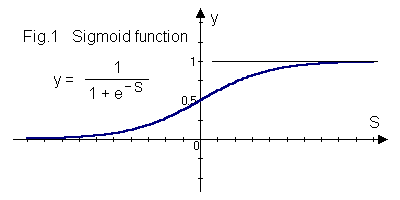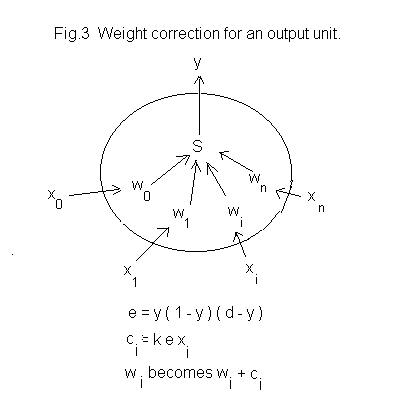# Neural Networks

## Introduction

Part I dealt with neural networks operating with fixed weights. That system works when it is possible to discriminate clearly between inputs and appropriate weights can be found for the network units. A network using learning by back-propagation can adjust weights to improve discrimination when input data is incomplete or noisy.

Weights are adjusted by calculating correction increments from a known input to the net and the desired output and the actual output. The mathematics is given here in revelatory fashion; anyone who wants the dirty detail can find it in Ref.2 for Part I.

## The Sigmoid Function

In Part I the output of a unit with fixed weights was found by applying a hardlimiting function to the weighted sum of the inputs. Correction increments for back-propagation are calculated from a derivative of a unit’s output but the hardlimiting function is not continuous and so not differentiable. A continuous, monotonic function, the sigmoid function illustrated at Fig.1, is used instead.The sigmoid output y of a unit in the hidden or output layer is

y = 1 / (1 + e-S )

where S is the same weighted sum as for a fixed weights unit. That output is, as before, delivered to all output units from a hidden unit or forms part of the network output from the output layer. Each input layer unit still delivers its input unchanged to every hidden layer unit.

The working scheme for a hidden or output unit is now as shown at Fig.2. Notice that binary outputs cannot occur; sigmoid outputs can only tend towards 0 or 1 so in any network application criteria for evaluating such output is needed.## Correction of output unit weights

The correction procedure is different for output and hidden units because, initially at least, desired values exist only for the output units. Output layer units are corrected first.

An input for which a desired network output is known is delivered to the network. That input, the hidden layer output and the network output are recorded.

For each output unit an error value

e = y ( 1 - y ) ( d - y )

is calculated where y is the unit’s actual output and d is it’s desired output. Then the correction increment for each i’th weight of the unit is

ci = k e xi

where k is a constant, the learning rate, 0 < k < 1, and xi is the input to the weight wi.

The new weight is obtained by adding ci to the old weight.## Correction of hidden unit weights

The procedure a hidden unit is as for an output unit except that the error value for a weight is calculated using a weighted sum of all the error values for the output layer. The description below uses the notation shown in Fig.4 where we are working with the i’th weight of the r’th hidden unit and error values e1 to em have been calculated for the m output units.

The weights of the output layer having been adjusted a weighted sum Wr of the error values for the output units is calculated. The weights used are those for the input from hidden unit r, giving

Wr = wr1 e1 + wr2 e2 + . . . + wrm em

which is used to calculate the error value er for hidden unit r,

er = y ( 1 - y ) Wr

and the correction increment for the i’th weight of hidden unit r is

ci = k er xi

where xi is the input to wi of unit r and k is the learning constant.## Programs

At this point it would be nice to present a learning program utilising back-propagation but unfortunately that program is not yet reliable. If you want to know why just look back at the last few paragraphs. It will appear, ed willing, in Part III with learning versions of the networks presented in Part I. For now I offer some other programs for back-propagation procedures.

Program ‘PREP’ is the same as the program of that name in Part I except that it controls number format. Enter data at the prompts to set up a net. The numbers of input, hidden and output units are stored as ‘Ino’, ‘Hno’ and ‘Ono’. The weights for hidden and output units are stored as arrays at ‘Hwts’ and ’Owts’ with each unit’s weights as a column vector, unit 1 in the first column, w0 at the top.

```« "PREP" DROP
RCLF -49 CF -50 CF
"Enter no. inputs" "" INPUT OBJ-> 'Ino' STO
"Enter no. hidden units" "" INPUT OBJ-> 'Hno' STO
1 Hno FOR j
"Enter weights 0 to " Ino + " for hidden unit " + j +
"" INPUT OBJ-> NEXT
Hno 'Ino+1' 2 ->LIST ->ARRY TRN 'Hwts' STO
"Enter no. outputs" "" INPUT OBJ-> 'Ono' STO
1 Ono FOR j
"Enter weights 0 to " Hno + " for output unit " + j +
"" INPUT OBJ-> NEXT
Ono 'Hno+1' 2 ->LIST ->ARRY TRN 'Owts' STO STOF
»```

Checksum # 9340h, 468.5 bytes.

Program ‘RUNIT’ operates whatever net has been set up by ‘PREP’. It is the same as the program of the same name in Part I except that it calls the sigmoid function instead of ‘hardlimit’, controls number format and saves layer inputs as row vectors ‘HXi’ and ‘OXi’ for when it is called by the learning program. A layer output is obtained as a row vector by multiplying the row vector of inputs and the array of layer weights. Enter input values at the prompt. Net output values are delivered as a list, using 1 FIX format, with the value from output unit 1 at the head. For more decimal places alter the FIX parameter in the last line.

```« "RUNIT" DROP
-49 CF -50 CF
1 "Enter inputs 1 to " Ino + "" INPUT
OBJ-> 1 'Ino+1' 2 ->LIST ->ARRY
DUP 'HXi' STO
Hwts * sigmoid
[ 1 ] 1 COL+
DUP 'OXi' STO
Owts * sigmoid
OBJ-> OBJ-> DROP SWAP DROP ->LIST
1 FIX
»```

Checksum # 3970h, 242.0 bytes.

Program ‘sigmoid’ is called by ‘RUNIT’. It takes a row vector of layer outputs, disassembles it, rotates the elements on the stack while applying the sigmoid function to each one and then reassembles the vector.

```« "sigmoid" DROP
OBJ-> OBJ-> DROP SWAP DROP -> c
« 1 c
START NEG EXP 1 + INV c ROLLD NEXT
1 c 2 ->LIST ->ARRY
»
»```

Checksum # 2016h, 95.0 bytes.

Program ‘VYOO’ enables inspection of net elements to see how learning is affecting them. The small screen is a problem. Each row of a weights display shows the weights of one unit in 1 FIX format, the first unit at the top and w0 at the left. For more decimal places alter the FIX parameter. If an array is too large for the screen then user must recall the matrix and use the matrix writer instead, in which case the weights of units are seen as column vectors, unit 1 at the left, w0 at the top.

```« "VYOO" DROP
FONT-> FONT6 ->FONT -49 -50 CF CF CLLCD
"Net configuration -"
Ono + " output units, " +
Hno + " hidden units, " +
Ino + " input units." + 3 DISP
{ { "NEXT" « CONT » } } TMENU
7 FREEZE -1 WAIT DROP
1 FIX CLLCD
“Last hidden input;” 3 DISP HXi sho 4 DISP
“Last output input;” 6 DISP OXi sho 7 DISP
7 FREEZE HALT CLLCD
"Hidden weights -" 1 DISP Hwts unrap
7 FREEZE HALT
CLLCD
"Output weights -" 1 DISP Owts unrap
7 FREEZE HALT STOF ->FONT
{ { “FINIS” « 2 MENU » } { “AGAIN” « VYOO » } } TMENU
»```

Checksum # 23DCh, 529.0 bytes.

Program ‘sho’ is called by ‘VYOO’ to display layer input values as lists.

`« “sho” DROP OBJ-> OBJ-> DROP SWAP DROP ->LIST »`

Checksum # B08Ah, 35.5 bytes.

Program ‘unrap’ is called by ‘VYOO’ to display layer weights.

```« “unrap” DROP TRN OBJ-> OBJ-> DROP -> r c
« r 1 FOR R ->STR 1. 'c-1'
START " " SWAP + + NEXT
c R 1. - * 1 + ROLLD -1 STEP
1 r
FOR R R 1 + DISP
NEXT
»
»```

Checksum # 133h, 158.5 bytes.

The source code for the programs in this article can be downloaded from Peter Gatenby’s web site at http://www.freenetpages.co.uk/hp/pvg or HPCC’s web site here